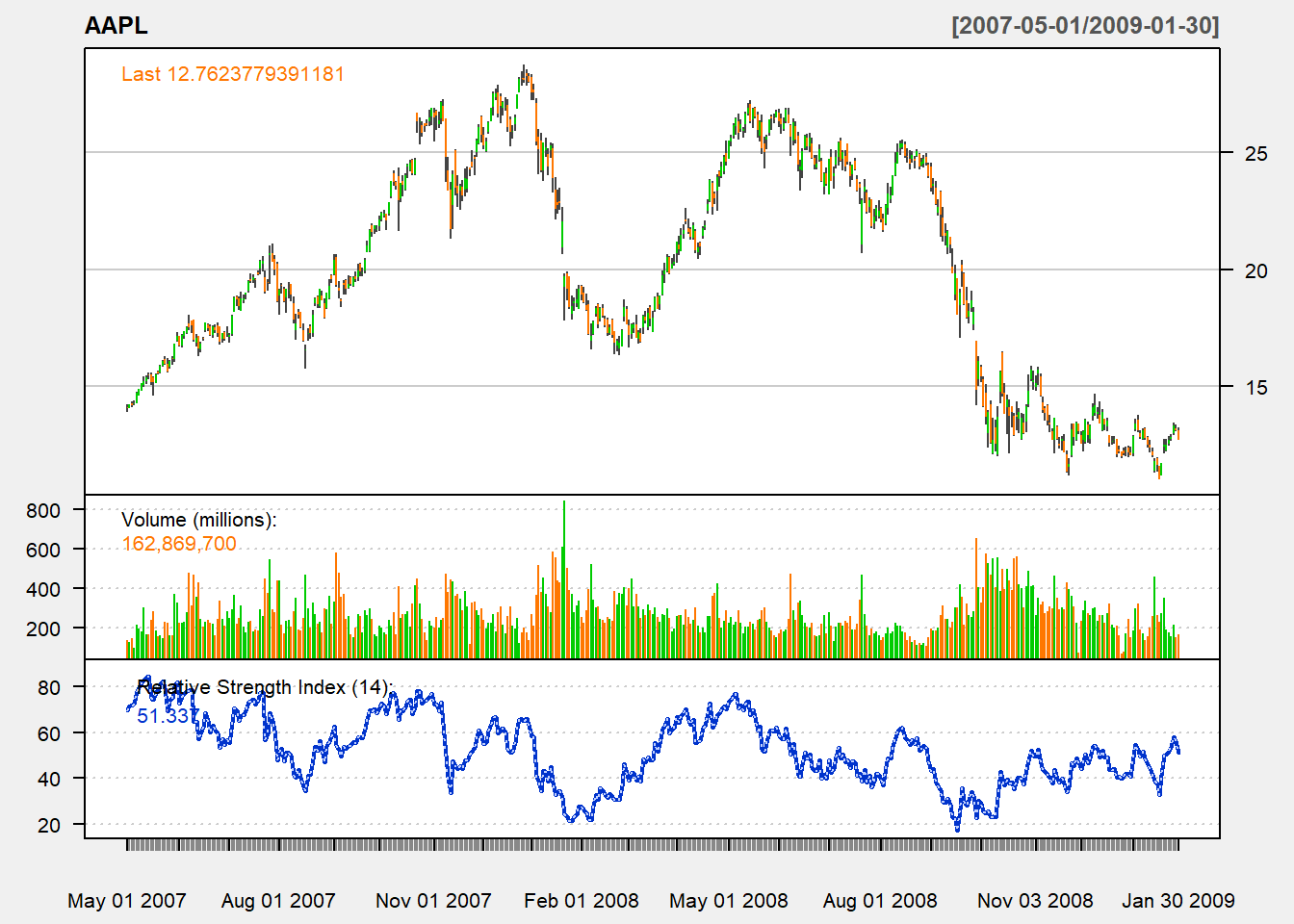## 5.11 TTR

In the TTR package, we can use RSI() function:

rsi <- RSI(Cl(AAPL), SMA, n=14)
tail(rsi,n=3)
##                 rsi
## 2012-12-26 38.85768
## 2012-12-27 35.28446
## 2012-12-28 38.26622

Note that the third input is a function. You may use SMA or EMA.

Further notice that this is different from what we have above. It is because their upward and downward indicators use numerical values instead:

$U_{t}=\left\{ \begin{array}{cl} P_t-P_{t-1}, & P_t>P_{t-1} \\ 0, & P_t \leq P_{t-1} \end{array} \right.$ and $D_{t}=\left\{ \begin{array}{cl} 0, & P_t\geq P_{t-1} \\ P_{t-1}-P_{t}, & P_t < P_{t-1} \end{array} \right.$

Hence, we need to modify our code:

myRSI <- function (price,n){
N <- length(price)
U <- rep(0,N)
D <- rep(0,N)
rsi <- rep(NA,N)
Lprice <- Lag(price,1)
for (i in 2:N){
if (price[i]>=Lprice[i]){
U[i] <- price[i]- Lprice[i]
} else{
D[i] <- Lprice[i]- price[i]
}
if (i>n){
AvgUp <- mean(U[(i-n+1):i])
AvgDn <- mean(D[(i-n+1):i])
rsi[i] <- AvgUp/(AvgUp+AvgDn)*100
}
}
rsi <- reclass(rsi, price)
return(rsi)
}

Now we can see that we have the same result:

rsi <- myRSI(Cl(AAPL), n=14)
tail(rsi,n=3)
##                [,1]
## 2012-12-26 38.85768
## 2012-12-27 35.28446
## 2012-12-28 38.26622

chartSeries(AAPL,
addRSI(n=14,maType="EMA")